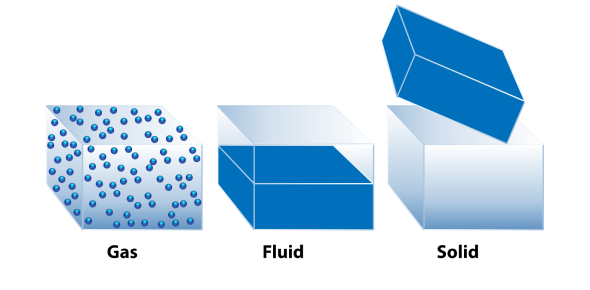# Properties Of Matter Quiz Exam!

15 Questions | Attempts: 420
ShareSettings.

• 1.
What is mass?
• A.

The amount matter an object takes up

• B.

A pure substance that has more than one value

• C.

The amount of space an object takes up

• 2.
Which measurement would be different if you were on the Moon versus on Earth?
• A.

Mass

• B.

Weight

• 3.
What is buoyancy?
• A.

The property of a substance that enables it to change its length, volume, or shape and to recover its original form

• B.

The ability to be easily changed into a new shape:

• C.

Is the force that causes objects to float.

• D.

Option 4

• 4.
What's malleability?
• A.

The property of a substance that enables it to change its length, volume, or shape and to recover its original form

• B.

The ability to be easily changed into a new shape

• C.

Is the force that causes objects to float.

• D.

Any

• 5.
What is elasticity?
• A.

The property of a substance that enables it to change its length, volume, or shape and to recover its original form

• B.

The ability to be easily changed into a new shape:

• C.

Is the force that causes objects to float.

• 6.
What is density?
• A.

The ability to be easily changed into a new shape:

• B.

The property of a substance that enables it to change its length, volume, or shape and to recover its original form

• C.

Is a measure of the mass of a substance per unit volume.

• 7.
You are given a mystery object in science class. Your teacher asks you to determine if your object is more or less dense than water. You place the object in the water and it sinks. What statement would best describe this situation?
• A.

Water is denser than the mystery substance.

• B.

They both have the same mass.

• C.

The mystery object must be less dense

• D.

The mystery object is denser than water.

• 8.
How is mass different than weight?
• A.

Mass and weight are not any different. They are exactly the same.

• B.

Mass is the amount of matter and wight is the amount of matter plus energy.

• C.

Mass is the amount of matter and weight is the amount of matter plus gravity.

• D.

​​​​​​Mass is the amount of matter and weight is the amount of matter plus electricity.

• 9.
Density is equal to which of the following?
• A.

Volume divided by mass

• B.

Mass plus volume

• C.

Mass divided by volume

• D.

​​​​​​Volume multiplied by mass

• 10.
What is the density of an object with a mass of 48 grams and a volume of 3 ml?
• A.

​​​​​​​144 g/ml

• B.

​​​​​​​16 g/ml

• C.

​​​​​​​45 g/ml

• D.

51 g/ml

• 11.
A piece of Silver has a mass of 77 grams and a volume of 11 cm3.  What is the density of Silver?
• A.

77 grams

• B.

11 cm3

• C.

​​​​​​​88 g/cm3

• D.

​​​​​​​7 g/cm3

• 12.
The object was placed in water to determine its volume.  Before it was placed in the water, there were 100 ml of water.  After it was submerged, 30 ml of water flowed out to the smaller container.  What is the volume of the object?
• A.

130 ml

• B.

100 ml

• C.

30 ml

• 13.
A 48-gram metal bar was placed in water.  The initial water level was 80 ml.  The final volume was 86 ml.  What are the volume of the bar and its density?
• A.

​​​​​​​Volume= 6 ml, density= 180 g/ml

• B.

​​​​​​​Volume = 6 ml, density= 0 g/ml

• C.

Volume= 6 ml, density= 8 g/ml

• D.

​​​​​​​Volume= 6 ml, density = 54 g/ml

• 14.
A 10 cm3 sample of metal has a mass of about 90 g. What is the density of the metal?
• A.

9 g/cm3

• B.

80 g/cm3

• C.

100 g/cm3

• D.

900 g/cm3

• 15.
Tick all the physical properties of matter.
• A.

Mass

• B.

Weight

• C.

Flammability

• D.

Buoyancy

• E.

Density

• F.

Elasticity

• G.

Reactivity

• H.

Volume

## Related TopicsBack to top
×

Wait!
Here's an interesting quiz for you.